## Entering formulas

In this lesson you can learn how to enter formulas in Excel.

Excel Spreadsheet Data to enter by selecting the cell and enter data directly in the cell or the formula bar. Signs of active line (3) and the active column (C) are on a darker background. The active cell has a bold border and its address is displayed in the name field.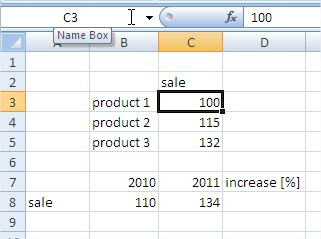If you want to go to one of the cells click it the left mouse button, drag the arrow keys or the active cell type in the name of the cells in the name field.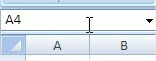Please set the active cell C6. Type a formula in that cell summation of the above 3 items for sale. This can be done in many ways, we will use the simplest sum of selecting the icon, which is located on the Ribbon: ‘Formula One’. Once it clicked, our sheet should look like is shown in the figure below.

## SUM Formula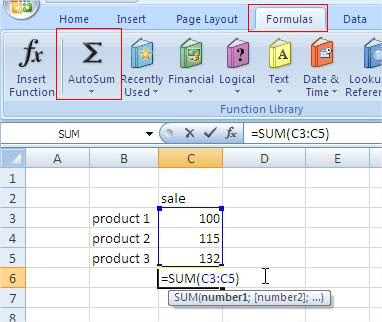Excel tries to guess what extent we want to sum up, in most cases, proposing appropriate. Range, which will be pooled is surrounded by a blue rectangle and typed in the formula sum (C3: C5). At the function gives the syntax of Excel functions sum, novice users can safely ignore this information. In this case, the range is correct, so we confirm it by pressing enter.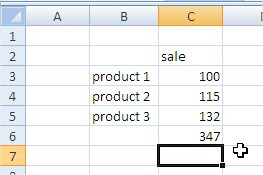The formula amount is entered, and activated the cell is located below. At any time we can re-enter this formula and verify that the proper range is totaled. Just double click the cell with the formula, which in this case will effect such as the following figure.## Percentage Increase Formula

The cells can enter the formula using mathematical marks and indicating the cells to be used in these calculations. It is important to begin by placing the formula =sign The following example was introduced formula that calculates the percentage increase in letters of division and subtraction, and pointing data, from which cells are to be used in the calculation. The formula for the percentage increase is a ‘new value’ / ‘old value’ -1.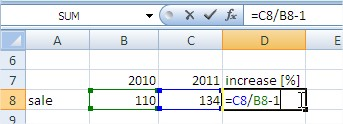But after entering the formula and press Enter.If sales figures are changed, the formula calculates the percentage increase automatically, and will indicate the new value. Excel does not always suggest the right solutions. Let this in two examples below. Introduce the sum in cell C11. We can do this by clicking on the amount, but faster would be to use the keyboard shortcut Alt + = or holding down the left Alt, hit the symbol =.As you can see Excel decided to sell the products to add also the year that is the column header. If the range proposed by Excel not responding to us, we can change it by clicking on one corner of the range and holding the left key or extend, you can also click drag the edge of the field holding the left mouse button to another location.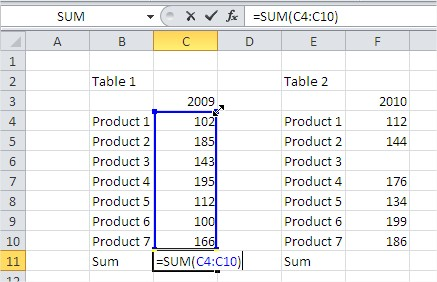That changed the formula, press Enter to confirm. We now type the sum of the Table 2, in cell F11.This time Excel has stopped on the first empty cell, grab the back corner of the area surrounded by a line and drag it to the right place. Tables 1 and 2 should show the following totals.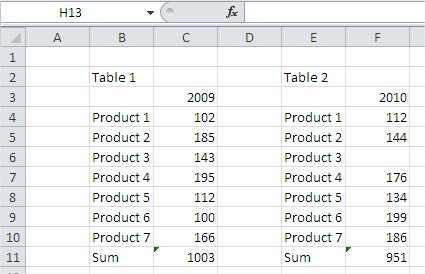## Template

```Further reading: